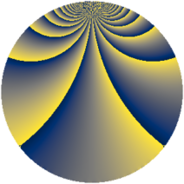# Properties

 Label 322.2.kLevel $322$ Weight $2$ Character orbit 322.k Rep. character $\chi_{322}(83,\cdot)$ Character field $\Q(\zeta_{22})$ Dimension $160$ Newform subspaces $2$ Sturm bound $96$ Trace bound $2$

# Related objects

## Defining parameters

 Level: $$N$$ $$=$$ $$322 = 2 \cdot 7 \cdot 23$$ Weight: $$k$$ $$=$$ $$2$$ Character orbit: $$[\chi]$$ $$=$$ 322.k (of order $$22$$ and degree $$10$$) Character conductor: $$\operatorname{cond}(\chi)$$ $$=$$ $$161$$ Character field: $$\Q(\zeta_{22})$$ Newform subspaces: $$2$$ Sturm bound: $$96$$ Trace bound: $$2$$ Distinguishing $$T_p$$: $$3$$

## Dimensions

The following table gives the dimensions of various subspaces of $$M_{2}(322, [\chi])$$.

Total New Old
Modular forms 520 160 360
Cusp forms 440 160 280
Eisenstein series 80 0 80

## Trace form

 $$160 q - 16 q^{4} + 8 q^{9} + O(q^{10})$$ $$160 q - 16 q^{4} + 8 q^{9} - 16 q^{16} - 20 q^{18} - 66 q^{21} + 36 q^{23} - 52 q^{25} - 22 q^{28} + 24 q^{29} + 44 q^{30} + 14 q^{35} + 8 q^{36} - 44 q^{37} - 16 q^{39} - 44 q^{43} + 50 q^{49} + 24 q^{50} - 44 q^{51} + 22 q^{56} - 44 q^{57} - 80 q^{58} + 110 q^{63} - 16 q^{64} - 308 q^{65} + 80 q^{70} - 16 q^{71} - 64 q^{72} + 126 q^{77} - 176 q^{79} + 36 q^{81} + 22 q^{84} + 60 q^{85} - 44 q^{86} - 8 q^{92} + 104 q^{93} + 64 q^{95} - 60 q^{98} + 88 q^{99} + O(q^{100})$$

## Decomposition of $$S_{2}^{\mathrm{new}}(322, [\chi])$$ into newform subspaces

Label Dim $A$ Field CM Traces $q$-expansion
$a_{2}$ $a_{3}$ $a_{5}$ $a_{7}$
322.2.k.a $80$ $2.571$ None $$-8$$ $$0$$ $$0$$ $$-11$$
322.2.k.b $80$ $2.571$ None $$8$$ $$0$$ $$0$$ $$11$$

## Decomposition of $$S_{2}^{\mathrm{old}}(322, [\chi])$$ into lower level spaces

$$S_{2}^{\mathrm{old}}(322, [\chi]) \cong$$ $$S_{2}^{\mathrm{new}}(161, [\chi])$$$$^{\oplus 2}$$